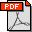Article Details

SIMULTANEOUS OPTIMIZATION OF HELMHOLTZ EQUATION OF STATE FOR R-600 AND R-600A

Oleh   KAN Koemleng 
 Kontributor / Dosen Pembimbing : Ir. I Made Astina, M.Eng., Ph.D.; Jenis Koleksi : S2 - Tesis Penerbit : FTMD - Teknik Mesin Fakultas : Fakultas Teknik Mesin dan Dirgantara (FTMD) Subjek : Engineering & allied operations Kata Kunci : hermodynamic equation; simultaneous optimization; Helmholtz equations Sumber : Staf Input/Edit : Alice Diniarti   Ena Sukmana File : 9 file Tanggal Input : 2020-05-04 08:48:24This research exihibits a simultaneous optimization of thermodynamic equation of state for Hydrocarbon refrigerants. New fundamental Helmholtz equations of state for natural refrigerants of R-600 and R-600a have been simultaneously established based on the new available experimental measurement in accordance with considering on the theoretical background. The aspect of combining genetic algorithm optimization method with weight least square regression analysis was assigned as a potential and efficient method to carry research objectives in this study. The thermodynamic property models for the fluids were previously developed by some researchers. The present models still have some problems. The absolute average deviation with respect to the new thermodynamic equations of state for R600, and R600a refrigerants are estimated to be 0.15 %, and 0.18 % in in liquid density; 0.32 %, and 0.81 % in gaseous density; 0.037 %, and 0.16 % in saturated-liquid density; 0.36 %, and 0.59 % in saturated-vapor density except at higher temperature; 0.31 %, and 0.46 % % in vapor pressure except at lower temperatures; 0.015 % , and 0.06 % in ideal-gas isobaric specific heat; 0.39 %, and 1.82 % in heat capacities; 0.08 %, and 0.018 in speed of sound in gaseous phase; 0.53 %, and 1.25 % in speed of sound in liquid phase respectively.

Cari
Artikel Terkait
Tautan